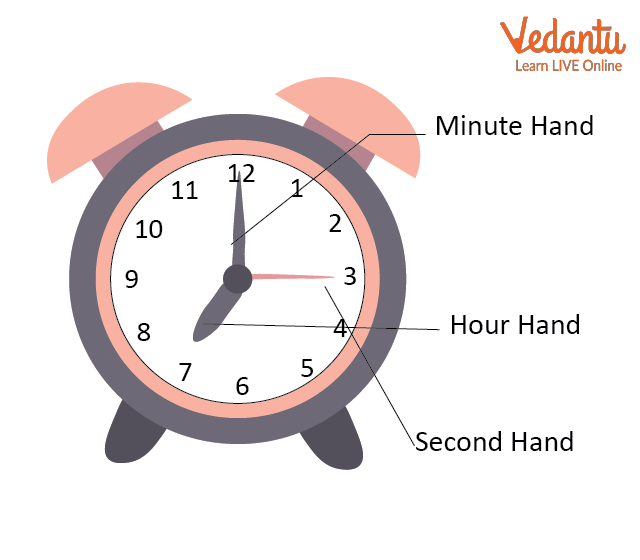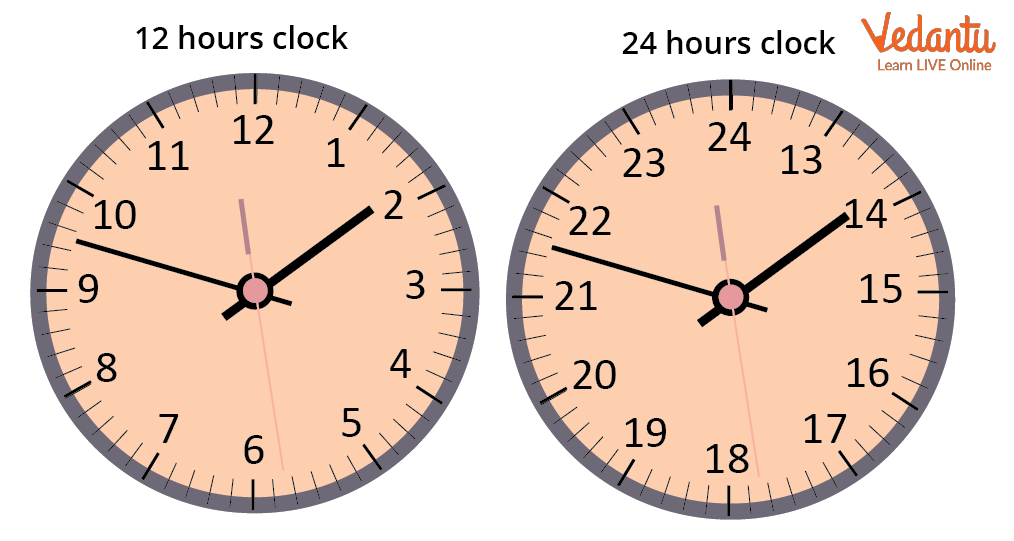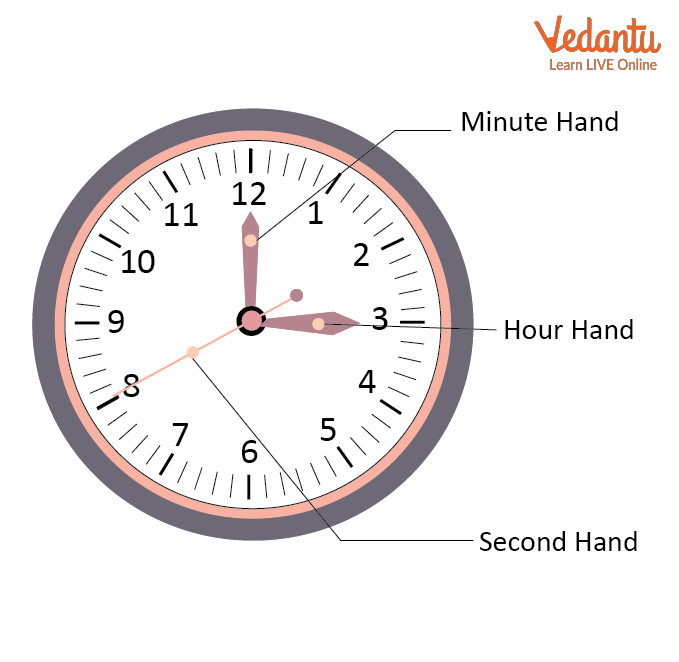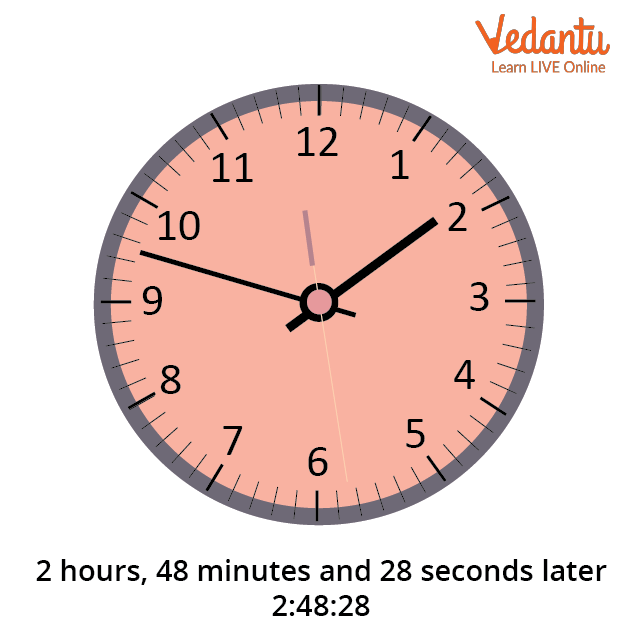Courses
Courses for Kids
Free study material
Free LIVE classes
More

# Seconds to Hours and Minutes ConversionLIVE
Join Vedantu’s FREE Mastercalss

## What Is Time?

We all have seen three hands on a clock face. The minute hand indicates the time in minutes, the hour hand indicates the time in hours, and the second hand shows the time in seconds. There are 12 central divisions on the clock, each lasting one hour, and five minor divisions spaced equally between them.

Five minutes are passed when the minute hand transitions from one large division to the subsequent large division. Each of the 12 significant divisions lasts five minutes, making the equation for an hour: 12 x 5 = 60 minutes.

Time is the eternal progression of past, present, and future events, indicating that time comprises every series of events that have occurred or will occur in the future. It is measured in minutes, seconds, and hours. The clock is used to measure time more precisely and accurately.

## What Is a Clock?

The clock is a device for measuring the time that displays the passage of hours, minutes, and seconds.Clock

The units used to measure time are the hour, minute, and second. The minute hand is the largest and fastest hand on the clock, followed by the second hand and the Hour hand.

## Time

Time is defined as the duration of all events or the specific instant that something occurs. Time includes all events that have occurred or will occur in the future.

Time is written as- Hour: Minutes: Seconds.

Example: 02: 48:28Time keeper

## Two Different Types of Clocks

• Clock with a 12-hour format: As the name implies, this clock displays time from 1 to 12. These clocks lack digital displays, and the wall clocks are an example.

• Clock in 24-hour format: This clock displays the time from 1 to 24 as its name says. These clocks are digital, and some wrist-watches, railway’s digital clocks, etc. are examples of such clock format.

In a 12-hour clock, the entire 24-hour period is divided into two segments, from midnight to noon and from midday to midnight. Ante Meridiem, or before noon, is what "A.M." stands for. As a result, we add the A.M. suffix to the time from midnight to noon. For instance, we might say that it is 9 am. Post Meridiem, or "afternoon," is what "P.M." stands for. As a result, we add the P.M. suffix to the end of the time from noon to midnight, for instance, 4 p.m.Hour, Minute, and Seconds Hands

## Seconds to Hours Formula

To convert from seconds to hours, divide the number of seconds by 3600.

For instance:

Convert 60 seconds in an hour.

As we know the seconds to hours formula, we need to divide the given time by 3600. Since, here we have 60 seconds, 60 divided by 3600 will give us 0.016 hours.

Conversions:

- 1 Hour = 60 Minutes

- 1 Minute =$\dfrac{1}{60}$ Hour

- 1 Minute = 60 Seconds

- 1 Second = $\dfrac{1}{60}$ Minutes

## How to Convert Seconds to Minutes

To change seconds to minutes, multiply the digit by 60. Simply reverse the process of converting minutes to seconds if you start from seconds and need to convert to minutes. To change secs to mins, divide by 60.

For instance:

30 secs divided by 60 mins equals 0.5 mins.

One minute and 30 seconds are identical to 90 seconds.

## How to Convert Minutes to Seconds

Keep in mind that one minute consists of 60 seconds. Remember the magic number: 60 seconds for every minute before you begin your conversion. It will be much simpler to convert if you keep this in mind.

For instance:

Change 5 min 37 sec into seconds.

Solution: 1 min = 60 sec

Thus, 5 min = 5 x 60 = 300 sec.

5 min 37 sec = 300 sec + 37 sec = 337 seconds

## Solved Examples

Example 1: Convert 270 minutes into seconds.

Ans: We know that one hour is equal to 60 minutes.

Additionally, we know that one minute equals 60 seconds.

Thus, 270 minutes equals 270 x 60, or 16,200 seconds.

Example 2: Convert 4800 seconds into hours.

Ans: First, we need to convert seconds into minutes and then minutes into hours.

$= 4800 \text{ seconds }= \left(4800 \div 60)\text{ minutes }(1\text{ second }=\dfrac{1}{60}\text{ minute}\right)$

$= 80$minutes

$= 80 \div 60$ hours $\left(\text{ minute }=\dfrac{1}{60}\text{ hour}\right)$

= 1 hour 20 minutes

Example 3: Convert 36000 seconds into hours.

Ans: First, we have to convert seconds into minutes and then minutes into hours.

$= 36000 \text{ seconds }= \left(3600 \div 60)\text{ minutes }(1\text{ second }=\dfrac{1}{60}\text{ minute}\right)$

$= 600$minutes

$= 600 \div 60$ hours $\left(\text{ minute }=\dfrac{1}{60}\text{ hour}\right)$

= 10 hoursHands of a Clock

Q1. How many minutes are in 600 seconds?

Ans: 10 minutes are in 600 seconds

Q2. Convert 7300 seconds to minutes to hours.

Ans: 7,300 Seconds = 2.0277 Hours = 2 Hours, 1 Minute and 39 Seconds.

## Summary

Time is the continued ongoing growth of past, present, and future scenes. It simply means every sequence of events that has happened, which is occurring or will happen, is created by time. It is estimated in hours, minutes, and seconds. The device used to measure time is known as a clock. The hour hand is the smallest and slowest in the watch, then comes the second hand, and the largest is the minute hand.

Last updated date: 26th Sep 2023
Total views: 115.2k
Views today: 2.15k

## FAQs on Seconds to Hours and Minutes Conversion

1. Who invented time and clock?

The Egyptians gave us the ancestor of our current hours by dividing the twelve-hour time from sunrise to sunset into twelve equal pieces. As one-twelfth of the daylight period, the Egyptian hour changed with the length of the day and as well as with the seasons rather than being a continuous quantity of time like it is now.

However, the inventor of the clock is typically recognised as Christiaan Huygens. He created the first pendulum-driven clock after formulating the relationship between pendulum length and time.

2. What do you understand by time and which hand is the longest in the clock?

Time is indefinite, the continued progress of past, present, and future events. Time is the progression of events from the past into the future. Time moves only in one direction. It's possible to move forward in time, but not backward. Scientists believe memory formation is the basis for human perception of time.

The minute hand is the most extended hand in the clock.

3. How many types of clocks are there?

The different types of clocks are as follows:

• Analog Clocks: These clocks have moving hands of an hour and minute handles over an arrangement of fixed numbered dials representing 12 hours of one day.

• Digital Clocks: It displayed time in 24-hour notation.

• Electronic Word Clocks: These clocks are not based on numeric displays, but instead, write natural sentences on the screen to tell us what time it is.

• Auditory Clocks: These clocks that tell time by recording human voice or computer generated voice.

• Tactile Clocks: Clocks that produce physical representations of the numbers on their surfaces, either as standard numbers or in blind text code.

• Multi Display Clocks: These clocks can be analog or digital, with the ability to display multiple time zones, have multiple faces, or use multiple time standards.